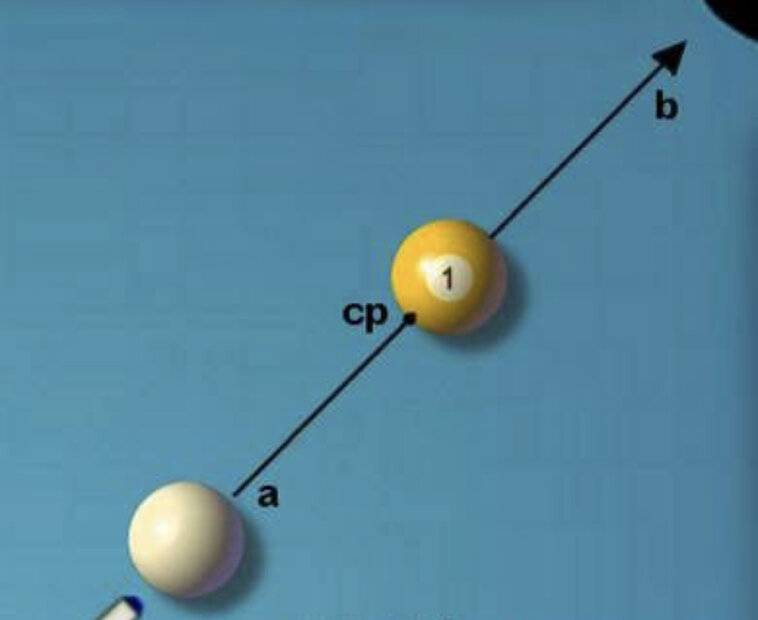# Pool Ball Simple Question 🎱

• B
Joe seki
TL;DR Summary
Can someone clear this up for me pleaseA=Cueball start position
CP=Centre Point where balls collide
B=The pocket

Hey guys, I was asked this question that seems completely obvious but I keep second guessing myself over it, I have included a basic picture to explain what I’m about to describe

Say you were to take the above pool shot, at just enough force for the yellow ball to reach B and fall into the pocket

How many more times of that force would be required if the balls were double in size?

My brain says it’s obviously just twice the force but considering there are 2 balls that will be increased in size I’m having doubts lol

Thanks in advance and sorry if this is a silly question 🙃

Joe Seki

Homework Helper
How many more times of that force would be required if the balls were double in size?
It depends.

For ideal pool balls on an ideal table, the question does not make sense since there is no minimum force that will do the job. Any force, no matter how small will be enough to make a pool ball roll an infinite distance.

So we must be talking about balls and tables that are not ideal.

In what way are they not ideal? You tell us.

Probably it will come down to how one models rolling resistance on felt.

•Delta2, sophiecentaur, Bystander and 2 others
Staff Emeritus
In what way are they not ideal? You tell us.
Exceptionally well said.•Delta2 and sophiecentaur
Homework Helper
Gold Member
Probably it will come down to how one models rolling resistance on felt.
I would also expect some rolling with slipping before the collision.

•sophiecentaur
Staff Emeritus
2022 Award
How many more times of that force would be required if the balls were double in size?

My brain says it’s obviously just twice the force but considering there are 2 balls that will be increased in size I’m having doubts lol
Assuming that doubling the size means to increase the diameter of the ball by 2x, then the mass of each ball would increase by a factor of 8 (volume increase from 4π/3 to 32π/3 if we set the initial radius to 1). Thus the mass is now 8x that of normal and far more than 2x force is needed. Especially if we look at the moment of inertia increase (formula below).

Assuming the balls increase in mass by 2x, and by size proportionally, the force necessary is probably somewhat more than 2x since friction on the balls has now increased due to the increased mass of the balls. Then there's the increased moment of inertia. So not only are the balls twice as hard to accelerate linearly, the are also harder to accelerate rotationally proportional to r2 since the moment of inertia of a solid sphere is given by l =2/5MR2.

•Delta2, sophiecentaur and PeroK
Homework Helper
also harder to accelerate rotationally proportional to ##r^2## since the moment of inertia of a solid sphere is given by ##l =2/5MR^2##
That part cancels out neatly. You double the radius and quadruple the moment of inertia, thus quadrupling kinetic energy. But you halve the angular velocity which quarters the kinetic energy. For a given linear velocity, rotational kinetic energy ends up proportional to mass alone. The radius is irrelevant.

If you look at angular momentum, force and acceleration, the cancellation still happens. This time the moment of inertia quadruples and the angular rotation rate halves, so angular momentum rises proportional to radius. But the moment arm of the pool cue (or of the felt converting slipping to rolling) increases as well.

Last edited:
•sophiecentaur
My brain says it’s obviously just twice the force but considering there are 2 balls that will be increased in size I’m having doubts lol
The number of balls is irrelevant.
The cue ball will stop on contact. So only one ball is moving at one time for an inline cannon, or carom shot.

•jbriggs444
Staff Emeritus
2022 Award
That part cancels out neatly. You double the radius and quadruple the moment of inertia, thus quadrupling kinetic energy. But you halve the angular velocity which quarters the kinetic energy. For a given linear velocity, rotational kinetic energy ends up proportional to mass alone. The radius is irrelevant.
My mistake then. Been a while since I took my physics 101 course.

Homework Helper
My mistake then. Been a while since I took my physics 101 course.
One of those counter-intuitive notions that one stores in the "cool things" corner of the brain.

"A sphere rolling down a slope will accelerate down at the same rate regardless of radius, but hollow it out and it'll accelerate at a different rate"

•Drakkith
Homework Helper
Gold Member
"A sphere rolling down a slope will accelerate down at the same rate regardless of radius, but hollow it out and it'll accelerate at a different rate"
And a can of chicken noodle soup will accelerate slower than an identical can that has been frozen. (I used to do this demo.)

Homework Helper
Gold Member
2022 Award
One of those counter-intuitive notions that one stores in the "cool things" corner of the brain.

"A sphere rolling down a slope will accelerate down at the same rate regardless of radius, but hollow it out and it'll accelerate at a different rate"
Gravity is geometry in more ways than one!

•